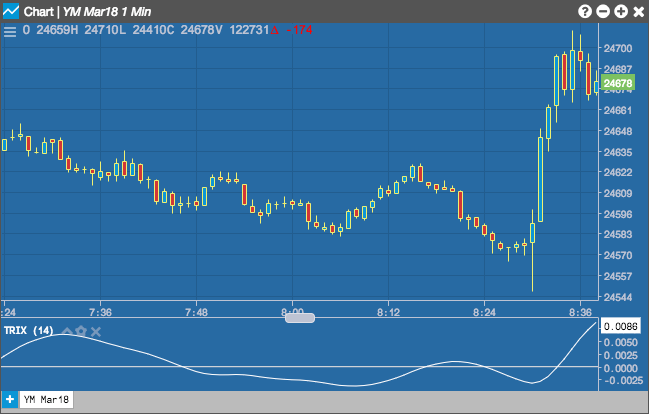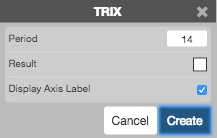Charts

# TRIX

The Triple Exponential Moving Average Oscillator (TRIX) by Jack Hutson is a momentum indicator that oscillates around zero. It displays the percentage rate of change between two triple smoothed exponential moving averages.## Configuration Options• Period: Number of bars to use in the calculations.
• Color Selectors: Colors to use for graph elements.
• Display Axis Label: Whether to display the most recent value on the Y axis.

## Formula

$EMA1 = EMA1_\text{n-1} + \left ( \left (\frac{2}{(n + 1)} \right) * (P_n - EMA1_\text{n-1}) \right)$

$EMA2 = EMA2_\text{n-1} + \left ( \left (\frac{2}{(n + 1)} \right) * (EMA1_n - EMA2_\text{n-1}) \right)$

$EMA3 = EMA3_\text{n-1} + \left ( \left (\frac{2}{(n + 1)} \right) * (EMA2_n - EMA3_\text{n-1}) \right)$

$TRIX = \frac{(EMA3_n - EMA3_\text{n-1})}{EMA3_\text{n-1}}$

where:

• Pn = the current price.
• EMA1n-1 = the exponential moving average value of n periods back
• EMA2n-1 = the exponential moving average value of n periods back
• EMA3n-1 = the exponential moving average value of n periods back